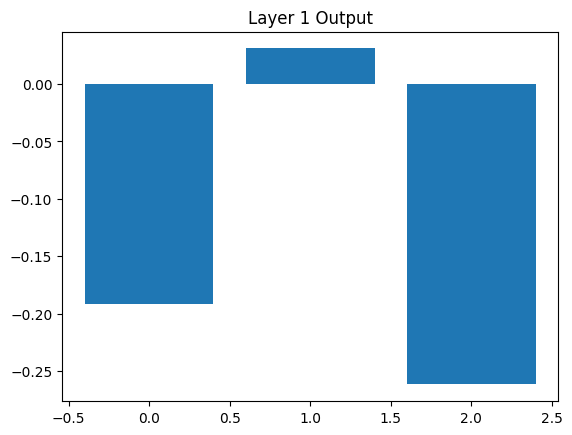# Iris prediction with neural network

Author

Haky Im

Published

January 1, 1999

Show the code
``````## conda install pytorch torchvision torchaudio cudatoolkit=<version> -c pytorch

## (test-env) MED-ML-4210:1999-01-01-iris-dataset-analysis haekyungim\$ conda install pytorch::pytorch torchvision torchaudio -c pytorch
## pip install scikit-learn ``````
Show the code
``````import torch
import torch.nn as nn
import torch.optim as optim
from sklearn.model_selection import train_test_split
from sklearn.preprocessing import StandardScaler``````
Show the code
``````# Load the iris dataset
X = iris.data
Y = iris.target``````
Show the code
``````# Split the data into training and testing sets
X_train, X_test, Y_train, Y_test = train_test_split(X, Y, test_size=0.2, random_state=42)``````
Show the code
``````# Standardize the features
scaler = StandardScaler()
X_train = scaler.fit_transform(X_train)
X_test = scaler.transform(X_test)``````
Show the code
``````# Convert the data to PyTorch tensors
X_train = torch.tensor(X_train, dtype=torch.float32)
Y_train = torch.tensor(Y_train, dtype=torch.long)
X_test = torch.tensor(X_test, dtype=torch.float32)
Y_test = torch.tensor(Y_test, dtype=torch.long)``````
Show the code
``````# Define the neural network architecture
class Net(nn.Module):
def __init__(self):
super(Net, self).__init__()
self.fc1 = nn.Linear(4, 10)
self.fc2 = nn.Linear(10, 10)
self.fc3 = nn.Linear(10, 3)

def forward(self, x):
x = torch.relu(self.fc1(x))
x = torch.relu(self.fc2(x))
x = self.fc3(x)
return x``````
Show the code
``````# Create an instance of the neural network
model = Net()``````
Show the code
``````# Define the loss function and optimizer
criterion = nn.CrossEntropyLoss()
Show the code
``````# Train the model
num_epochs = 100
batch_size = 16

for epoch in range(num_epochs):
running_loss = 0.0
for i in range(0, X_train.size(0), batch_size):
inputs = X_train[i:i+batch_size]
labels = Y_train[i:i+batch_size]

# Forward pass
outputs = model(inputs)
loss = criterion(outputs, labels)

# Backward pass and optimization
loss.backward()
optimizer.step()

running_loss += loss.item()

if (epoch+1) % 10 == 0:
print(f"Epoch {epoch+1}: Loss = {running_loss:.4f}")``````
``````Epoch 10: Loss = 7.9727
Epoch 20: Loss = 5.2169
Epoch 30: Loss = 3.1653
Epoch 40: Loss = 2.2043
Epoch 50: Loss = 1.5700
Epoch 60: Loss = 1.1596
Epoch 70: Loss = 0.9131
Epoch 80: Loss = 0.7656
Epoch 90: Loss = 0.6722
Epoch 100: Loss = 0.6093``````
Show the code
``````# Evaluate the model on the test set
model.eval()
outputs = model(X_test)
_, predicted = torch.max(outputs, 1)
accuracy = torch.sum(predicted == Y_test).item() / Y_test.size(0)
print(f"Test Accuracy: {accuracy*100:.2f}%")``````
``Test Accuracy: 100.00%``
Show the code
``````#import torch
#import torch.nn as nn
import matplotlib.pyplot as plt

# Assuming you have an input tensor 'input_tensor'
input_tensor = torch.tensor([1, 2, 3, 4], dtype=torch.float32)

# Create an instance of the 'Net' model
model = Net()

# Set the model to evaluation mode
model.eval()

# Store the intermediate layer outputs
outputs = []

# Forward pass and store intermediate outputs
def hook(module, input, output):
outputs.append(output)

# Register the hook to capture intermediate outputs
hook_handle = model.fc3.register_forward_hook(hook)
model(input_tensor.unsqueeze(0))
hook_handle.remove()

# Plot the intermediate outputs
for i, output in enumerate(outputs):
print(i)
plt.figure()
plt.title(f'Layer {i+1} Output')
plt.bar(range(output.size(-1)), output.squeeze().detach().numpy())
plt.show()``````
``0``Show the code
``enumerate(outputs)``
``<enumerate at 0x28f870680>``
Show the code
``````
outputs``````
``[tensor([[-0.1914,  0.0311, -0.2617]], grad_fn=<AddmmBackward0>)]``